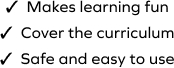# Subtract 10 and 100 from 3-Digit Numbers

SplashLearn - The complete PreK - Grade 5 Math & ELA Learning Program Built for Your Child
Home > Subtract 10 and 100 from 3-Digit Numbers
Help your child become fluent in subtraction with the help of this super fun game. The game requires students to complete a set of challenging tasks, and the students develop fluency with the concepts of subtraction in the process. Students subtract 10 and 100 from the same number in a set and observe the pattern in the difference. The game consists of problems of different structures, and by solving them, the students gain fluency in subtraction.